# Tutorial on English stress detection

This tutorial demonstrates the basics of English stress detection.

## Preprocessing

Before we start, let's add necessary toolboxes to the search path of MATLAB:

```addpath d:/users/jang/matlab/toolbox/utility
```

All the above toolboxes can be downloaded from the author's toolbox page. Make sure you are using the latest toolboxes to work with this script.

For compatibility, here we list the platform and MATLAB version that we used to run this script:

```fprintf('Platform: %s\n', computer);
fprintf('MATLAB version: %s\n', version);
fprintf('Script starts at %s\n', char(datetime));
scriptStartTime=tic;	% Timing for the whole script
```
```Platform: PCWIN64
MATLAB version: 8.5.0.197613 (R2015a)
Script starts at 04-Feb-2017 21:03:16
```

## Dataset construction

First of all, we shall collect all the audio files from the corpus directory. Note that

• The audio files have extensions of "au".
• For each audio file, we need to perform forced alignment in order to identify each phone within a given utterance.
```trainingDataDir='d:\dataSet\Merriam_Webster-2010-英語單字錄音';
if ~exist('auSet.mat')
myTic=tic;
auSet = rmfield(auSet, {'date', 'bytes', 'isdir', 'datenum'});
for i=1:length(auSet)
fprintf('%d/%d: file=%s\n', i, length(auSet), auSet(i).path);
auSet(i).error=0;
[parentDir, mainName]=fileparts(auSet(i).path);
items=split(mainName, '_');
if length(items)~=3
auSet(i).error=1;
auSet(i).errorMsg='Wrong format of file name ';
continue;
end
auSet(i).text=items{1};
auSet(i).sylNum=eval(items{2});
auSet(i).stressPos=eval(items{3});
if auSet(i).sylNum==1
auSet(i).error=1;
auSet(i).errorMsg='Single-syllable word';
continue;
end
try
asraOutput=waveAssess(auSet(i).path, auSet(i).text, 'english', 0, 'temp.pv');	% Forced alignment
auSet(i).asraOutput=asraOutput.cm.word;
catch
auSet(i).error=1;
auSet(i).errorMsg='Error in waveAssess';
end
end
% === Label vowel
vowelList = textread('data/vowelphone.txt', '%s', 'delimiter', '\n', 'whitespace', '');
for i=1:length(auSet)
for j=1:length(auSet(i).asraOutput)
phoneList=split(auSet(i).asraOutput(j).name, '_');		% List of phones
for k=1:length(auSet(i).asraOutput(j).phone)
auSet(i).asraOutput(j).phone(k).isVowel=sum(strcmp(phoneList{k}, vowelList));
end
end
end
fprintf('Total time for waveAssess: %g sec\n', toc(myTic));
fprintf('Saving auSet to auSet.mat...\n');
save auSet auSet
else
end
```
```Loading auSet.mat...
```

## More error checking

Here we shall perform more error checking to eliminate illegal entries, such as

• Utterances of single-syllable words
• Utterances which cannot be decoded correctly with the right number of syllables
```fprintf('Checking data for syllable consistence...\n');
for i=1:length(auSet)
if auSet(i).sylNum<2, auSet(i).errorMsg='Single-syllable word'; auSet(i).error=1; continue; end
if length(auSet(i).asraOutput)<2, auSet(i).errorMsg='ASRA error'; auSet(i).error=1; continue; end
if auSet(i).sylNum~=sum([auSet(i).asraOutput(2).phone.isVowel]), auSet(i).errorMsg='Wrong number of syllables'; auSet(i).error=1; continue; end
if auSet(i).stressPos>auSet(i).sylNum, auSet(i).errorMsg='Stress position larger than syllable number'; auSet(i).error=1; continue; end
for j=1:length(auSet(i).asraOutput)
phone=[auSet(i).asraOutput.phone];	% Concat all phones
vowelNum=sum([phone.isVowel]);		% No of vowels in this utterance
end
if vowelNum ~= auSet(i).sylNum	   % data inconsistency occurs!!! skip this one
auSet(i).errorMsg='No. of decoded vowels is different from no. of syllables';
auSet(i).error=1;
end
%	if i==21713, keyboard; end
end
fprintf('Deleting %d entries from auSet...\n', sum([auSet.error]));
auSet(find([auSet.error])) = [];
fprintf('Leaving %d entries in auSet...\n', length(auSet));
```
```Checking data for syllable consistence...
Deleting 3402 entries from auSet...
Leaving 13999 entries in auSet...
```

## Feature extraction from vowels

```if ~exist('auSet2.mat')
myTic=tic;
feaOpt=sdFeaExtract('defaultOpt');
for i=1:length(auSet)
fprintf('%d/%d\n', i, length(auSet));
auSet(i).input=sdFeaExtract(auSet(i), feaOpt);		% Features for vowel
auSet(i).output=ones(1, size(auSet(i).input, 2));	% 2 for stressed, 1 for unstressed
auSet(i).output(auSet(i).stressPos)=2;
end
fprintf('Total time for feature extraction: %g sec\n', toc(myTic));
fprintf('Saving auSet to auSet2.mat...\n');
save auSet2 auSet
else
end
```
```Loading auSet2.mat...
```

## Stress detection for 3-syllable words

```auSet=auSet([auSet.sylNum]==3);
ds.input=[auSet.input];
ds.output=[auSet.output];
ds.inputName=sdFeaExtract('inputName');
ds.outputName={'Unstressed', 'Stressed'};
```

Some of the input is nan, which needs to be removed first.

```ds.input(isnan(ds.input))=0;
```

## Dataset visualization

Once we have every piece of necessary information stored in "ds", we can invoke many different functions in Machine Learning Toolbox for data visualization and classification.

For instance, we can display the size of each class:

```figure;
[classSize, classLabel]=dsClassSize(ds, 1);
```
```19 features
13116 instances
2 classes
```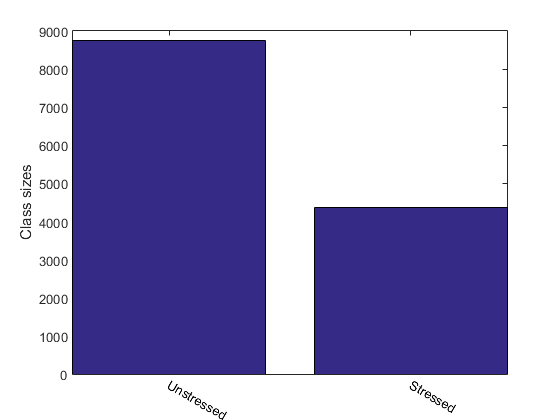We can plot the range of features of the dataset:

```figure; dsRangePlot(ds);
```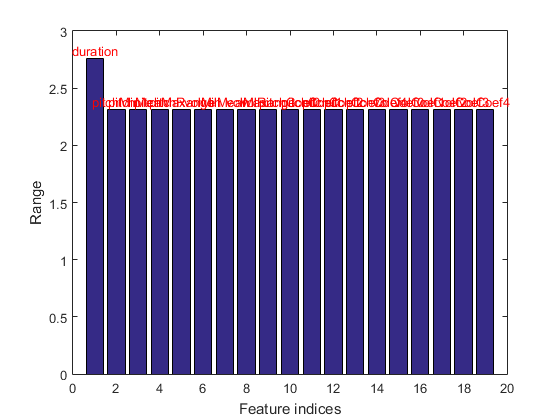We can plot the feature vectors within each class:

```figure; dsFeaVecPlot(ds);
```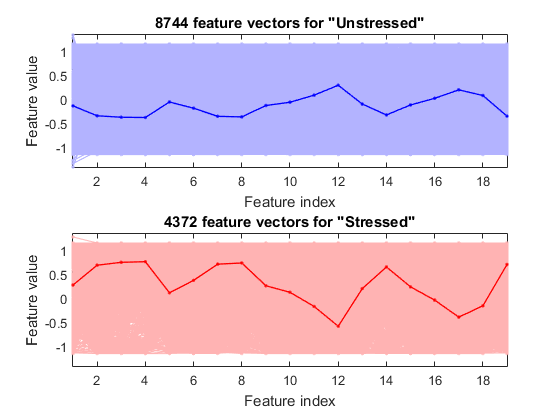## Dimensionality reduction

The dimension of the feature vector is quite large:

```dim=size(ds.input, 1)
```
```dim =

19

```

We shall consider dimensionality reduction via PCA (principal component analysis). First, let's plot the cumulative variance given the descending eigenvalues of PCA:

```[input2, eigVec, eigValue]=pca(ds.input);
cumVar=cumsum(eigValue);
cumVarPercent=cumVar/cumVar(end)*100;
figure; plot(cumVarPercent, '.-');
xlabel('No. of eigenvalues');
ylabel('Cumulated variance percentage (%)');
title('Variance percentage vs. no. of eigenvalues');
```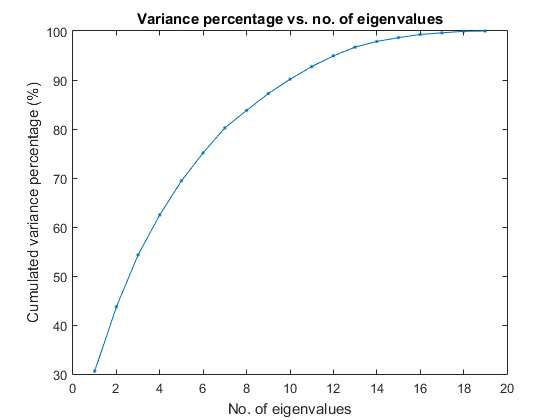A reasonable choice is to retain the dimensionality such that the cumulative variance percentage is larger than a threshold, say, 95%, as follows:

```cumVarTh=95;
index=find(cumVarPercent>cumVarTh);
newDim=index(1);
ds2=ds;
ds2.input=input2(1:newDim, :);
fprintf('Reduce the dimensionality to %d to keep %g%% cumulative variance via PCA.\n', newDim, cumVarTh);
```
```Reduce the dimensionality to 13 to keep 95% cumulative variance via PCA.
```

However, our experiment indicates that if we use PCA for dimensionality reduction, the accuracy will be lower. As a result, we shall keep all the features for further exploration.

In order to visualize the distribution of the dataset, we can project the original dataset into 2-D space. This can be achieved by LDA (linear discriminant analysis):

```ds2d=lda(ds);
ds2d.input=ds2d.input(1:2, :);
figure; dsScatterPlot(ds2d); xlabel('Input 1'); ylabel('Input 2');
title('Features projected on the first 2 lda vectors');
```
```Warning: Some of the eigenvectors are not real in lda!
```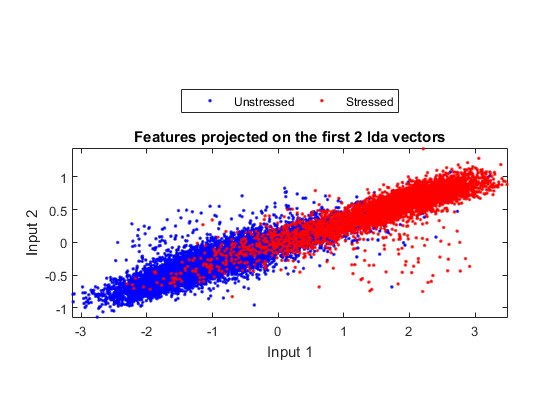Apparently the separation among classes is not obvious. This indicates that either the features or LDA are not very effective.

## Vowel-based classification

We can try the most straightforward KNNC (k-nearest neighbor classifier):

```[rr, computed]=knncLoo(ds);
fprintf('rr=%g%% for the original ds\n', rr*100);
ds2=ds; ds2.input=inputNormalize(ds2.input);
[rr2, computed]=knncLoo(ds2);
fprintf('rr=%g%% for ds after input normalization\n', rr2*100);
```
```rr=89.0363% for the original ds
rr=89.0439% for ds after input normalization
```

We can also try SVM classifier:

```myTic=tic;
sdOpt=sdOptSet;
if sdOpt.useInputNormalize, ds.input=inputNormalize(ds.input);	end		% Input normalization
cvPrm=crossValidate('defaultOpt');
cvPrm.foldNum=sdOpt.foldNum;
cvPrm.classifier=sdOpt.classifier;
plotOpt=1;
figure; [tRrMean, vRrMean, tRr, vRr, computedClass, cvData]=crossValidate(ds, cvPrm, plotOpt);
fprintf('Time for cross-validation = %g sec\n', toc(myTic));
```
```Warning: Name is nonexistent or not a directory:
d:\users\jang\matlab\toolbox\machineLearning\externalTool\libsvm-3.11\matlab
Fold = 1/10
Fold = 2/10
Fold = 3/10
Fold = 4/10
Fold = 5/10
Fold = 6/10
Fold = 7/10
Fold = 8/10
Fold = 9/10
Fold = 10/10
Training RR=94.49%, Validating RR=92.39%, classifier=svmc, no. of folds=10
Time for cross-validation = 37.7107 sec
```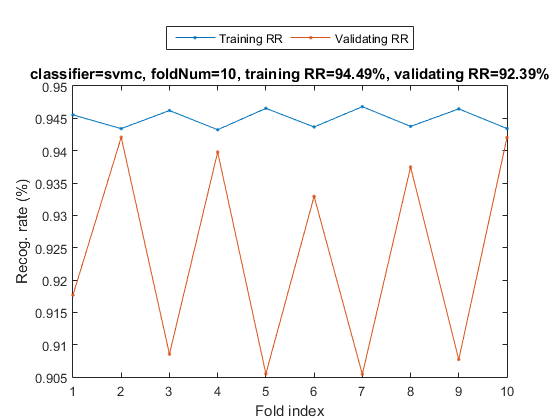The recognition rate of SVM is a little bit higher than KNNC.

To plot the confusion matrix, we need the validating output (and their corresponding indices) from each fold:

```computed=[];
vsIndex=[];
for i=1:length(computedClass)
computed=[computed, computedClass{i}];
vsIndex=[vsIndex, cvData(i).VS.index];
end
```

Now we can plot the confusion matrix as follows:

```desired=ds.output(vsIndex);
confMat = confMatGet(desired, computed);
cmOpt=confMatPlot('defaultOpt');
cmOpt.className=ds.outputName;
confMatPlot(confMat, cmOpt);
```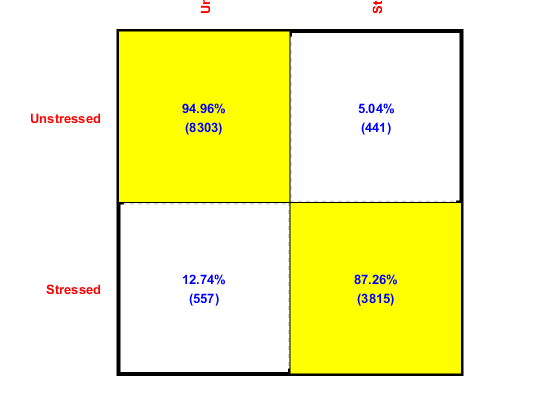## Summary

This is a brief tutorial on stress detection for English word pronunciation. There are several directions for further improvement:

• Explore other features and feature selection mechanisms.
• Explore other classifiers and their combinations.
• Explore other ways of combining vowel classifications to generate stress detection

## Appendix

List of functions and datasets used in this script

Date and time when finishing this script:

```fprintf('Date & time: %s\n', char(datetime));
```
```Date & time: 04-Feb-2017 21:05:10
```

Overall elapsed time:

```toc(scriptStartTime)
```
```Elapsed time is 114.002214 seconds.
```

Date and time when finishing this script:

```fprintf('Date & time: %s\n', char(datetime));
```
```Date & time: 04-Feb-2017 21:05:10
```